##Involutive homographic relation II

Consider a circle bundle, for example the bundle (I) of circles (c) orthogonal to two fixed circles c1 and c2. Consider also an arbitrary line e. For each point x on the line there is a circle c(x) of bundle (I) passing through x. Let y be the second intersection point of c(x) with the line. Here we identify points on the line e with their line coordinate (with respect to an arbitrary line-coordinate-system). The correspondence y=F(x) is an involutive homography relation between x and y. The image shows the graph of this relation, which as expected is a rectangular hyperbola symmetric with respect to the first diagonal. The fixed points of the involution (if any) correspond to the circles of bundle (I) which are tangent to the line e. On the graph they define its intersection points with the first diagonal.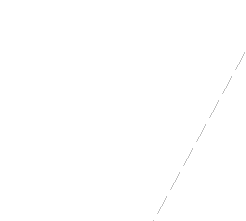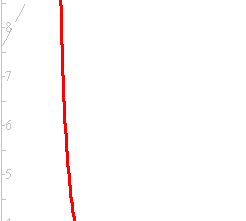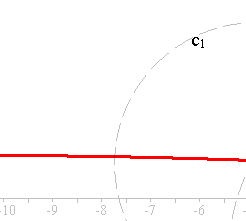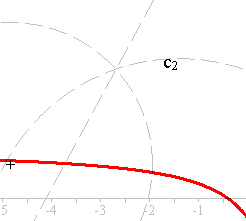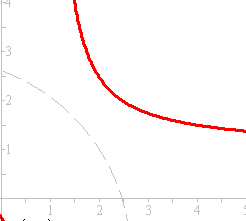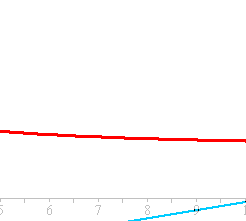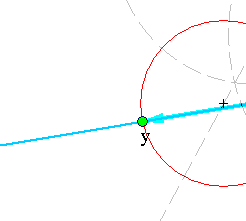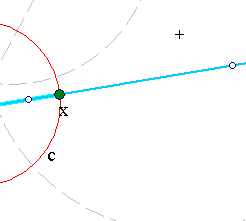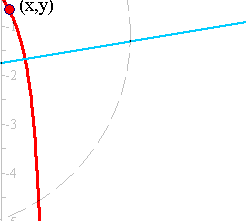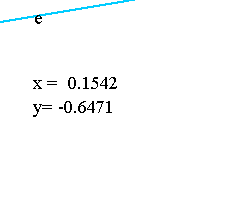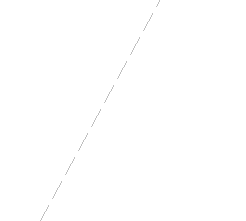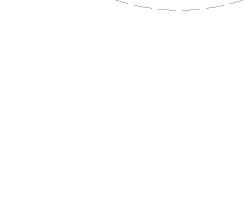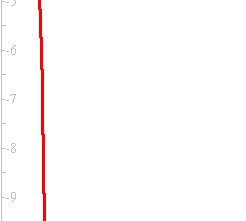This procedure, to define an involution out of a circle-bundle (and an arbitrary line) is inverse to the procedure examined in Involution.html , where we created a circle bundle out of an involution.

### See Also

CircleBundles.html
HomographicRelation.html
Involution.html
Involution3.html

 Produced with EucliDraw© http://users.math.uoc.gr/~pamfilos/